Previous: 4.6.3.1 Parallelization Strategy Up: 4.6.3 Parallelization Method Next: 4.6.3.3 Simulation Flow

### 4.6.3.2 Optimized Distribution Scheme

To guarantee an approximately deterministic behavior of the simulation and to avoid that slaves process ions which belong to different time steps, all slaves are synchronized by the master process after each time step. The simulation time required for one time step is therefore determined by the slowest slave, because all faster slaves are idle until the end of the time step. Therefore care has to be taken that all slaves require approximately the same simulation time for one time step.

The entrance points of the ions into the simulation domain are equally distributed over the surface of the simulation domain. Thus mainly the size of the scope of responsibility determines the simulation time, which makes the distribution of the subdomains the most critical task concerning the performance gain of the parallelization method. In case of a poor distribution the average idle times of the slaves can be of the order of the total simulation time and no performance gain is achieved by the parallelization as shown in Fig. 4.23. Several problem arise due to this requirement.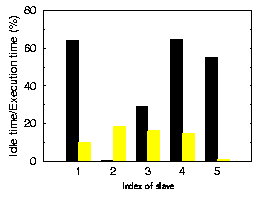If the simulation is performed on a cluster of workstations the simulation time is influnced by two additional parameters, the performance of a processor and its actual load, because normally a cluster is composed of processors with different CPU performances and the processors are not exclusively dedicated to a certain simulation run.

Worth mentioning is that even if all these parameters could be optimally considered when determining a distribution scheme it is never possible to reduce the average idle time to zero due to the statistical nature of the simulation problem.

An additional problem arises due to spatially varying material properties in the simulation doamin which require the application of different models and algorithms. Thereby the simulation time significantly depends on the entrance position of an ion into the simulation domain and the actual simulation time of each slave can only be determined during the simulation. The initial subdomain distribution can only be a more or less good guess.

To fully benefit from the parallelization strategy the communication overhead has to be minimized by choosing a distribution scheme with a minimum interface area between the slaves, because communication only occurs if an ion moves into the vicinity of an interface. The optimal situation would be if the scopes of responsibility of all slaves where prism with a square base, but this is generally not feasible.

The parallelization method makes use of a two step concept when distributing the subdomains. For the initial distribution it is assumed that the trajectory calculation time is constant in all subdomains. Due to the fact that the starting positions of the ions are equally distributed among the subdomains, the number of subdomains belonging to a certain slave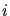can be estimated by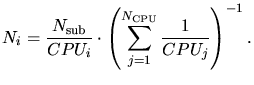(4.13)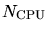is the number of available CPUs,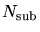is the total number of subdomains and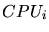is the performance factor of slave, which is proportional to the average time a slave needs to calculate one single ion trajectory. Starting with the fastest slave and continuing with slaves with decreasing speed the subdomains are distributed in the following way:
First a subdomain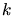is selected with a maximum distance from already distributed subdomains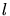. This is done by assuming a repulsive potential between subdomains belonging to different slaves and selecting the subdomainso that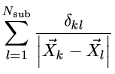(4.14)

becomes a minimum.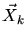and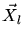are the coordinates of the centers of subdomainsand.is the Kronecker tensor.

Subdomains are moved to a slave by selecting the subdomain with the largest number of interfaces to the slave. If several subdomains meet this requirement the subdomain with the lowest energy assuming a repulsive potential between subdomains belonging to different slaves and an attractive potential between subdomains belonging to the same slave is selected. Thereby the average aspect ratio of the resulting scope of responsibility is kept low. Fig. 4.24 shows a possible subdomain distribution of 63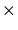63 subdomains on a workstation cluster consisting of 19 CPUs with four different CPU speeds.As will be explained more in detail in Sec. 4.6.3 the performance of each slave, considering the load and the variation of the physical properties, can be measured during the calculation of the first time step. Using (4.13) the number of subdomains can be optimized after finishing the first time step. In order to maximize the performance gain the distribution is adapted based on the initial distribution, because only small modifications of the initial distribution scheme correctly consider spatial variations in the simulation time. In this way subdomains are successively moved from slaves with small idle times to slaves with large idle times until an optimized distribution scheme for the simulation is attained.

A. Hoessiger: Simulation of Ion Implantation for ULSI Technology